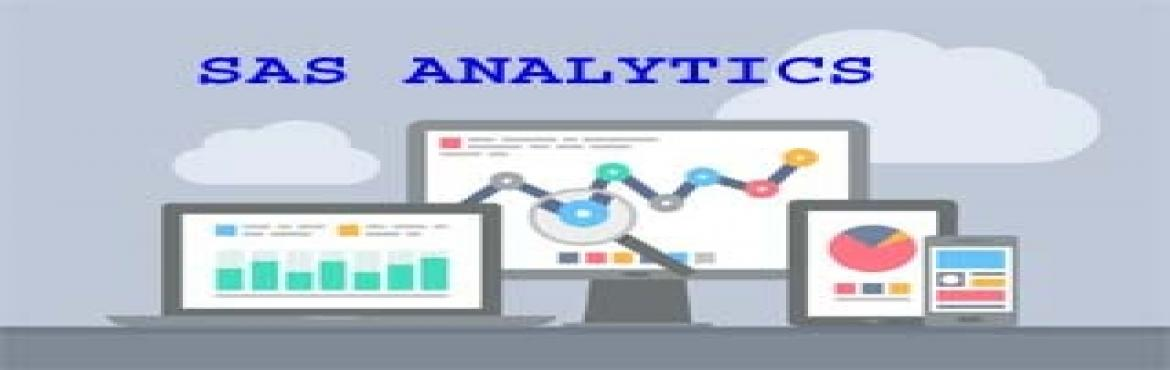# SAS Analytics Training

• ## Sas analytics

Sale Date Ended

INR 14400
Sold Out

#### Invite friends

Page Views : 46

SAS Analytics training

1. Variables
2. Data types
3. Scaling Techniques
4. Graphs & Frequency Distributions
5. Measures of Central Tendency,
6. Measures of Dispersion
7. Skew ness and Kurtosis,
8. Probability
9. Random Variable and Expectations
10. Probability Distributions Continuous and Discrete Distributions
11. Estimations
12. Sampling Theory Probability and Non Probability Sampling
13. Inferential Statistics Hypothesis Testing Parametric and Non Parametric
14. ANOVA and ANCOVA
15. Correlation Analysis

1. Regression Analysis Simple Regression, Multiple Regression]
2. Logistic Regression,
3. Factor Analysis
4. Cluster Analysis
5. Discriminant Analysis
6. Decision Trees[CHAID Analysis
7. CART]Forecasting techniques MA,AR, ARMA, ARIMA

All these techniques with Real Time Projects on Excel, SAS, SAS E Miner, R Studio.

Project Domains: Capital Market, Banking, Insurance.